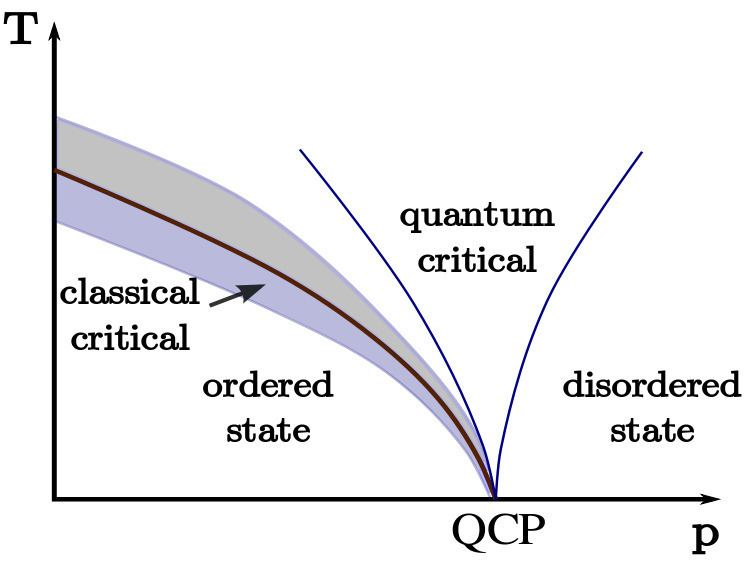# Luttinger liquid

Updated onA Tomonaga-Luttinger liquid, more often referred to as simply a Luttinger liquid, is a theoretical model describing interacting electrons (or other fermions) in a one-dimensional conductor (e.g. quantum wires such as carbon nanotubes). Such a model is necessary as the commonly used Fermi liquid model breaks down for one dimension.

## Contents

The Tomonaga-Luttinger liquid was first proposed by Tomonaga in 1950. The model showed that under certain constraints, second-order interactions between electrons could be modelled as bosonic interactions. In 1963, Luttinger reformulated the theory in terms of Bloch sound waves and showed that the constraints proposed by Tomonaga were unnecessary in order to treat the second-order perturbations as bosons. But his solution of the model was incorrect; the correct solution was given by Mattis and Lieb 1965.

## Theory

Luttinger liquid theory describes low energy excitations in a 1D electron gas as bosons. Starting with the free electron Hamiltonian:

H = k ϵ k c k c k

is separated into left and right moving electrons and undergoes linearization with the approximation ϵ k ± v F ( k k F ) over the range Λ :

H = k = k F Λ k F + Λ v F k ( c k R c k R c k L c k L )

Expressions for bosons in terms of fermions are used to represent the Hamiltonian as a product of two boson operators in a Bogoliubov transformation.

The completed bosonization can then be used to predict spin-charge separation. Electron-electron interactions can be treated to calculate correlation functions.

## Features

Among the hallmark features of a Luttinger liquid are the following:

• The response of the charge (or particle) density to some external perturbation are waves ("plasmons" - or charge density waves) propagating at a velocity that is determined by the strength of the interaction and the average density. For a non-interacting system, this wave velocity is equal to the Fermi velocity, while it is higher (lower) for repulsive (attractive) interactions among the fermions.
• Likewise, there are spin density waves (whose velocity, to lowest approximation, is equal to the unperturbed Fermi velocity). These propagate independently from the charge density waves. This fact is known as spin-charge separation.
• Charge and spin waves are the elementary excitations of the Luttinger liquid, unlike the quasiparticles of the Fermi liquid (which carry both spin and charge). The mathematical description becomes very simple in terms of these waves (solving the one-dimensional wave equation), and most of the work consists in transforming back to obtain the properties of the particles themselves (or treating impurities and other situations where 'backscattering' is important). See bosonization for one technique used.
• Even at zero temperature, the particles' momentum distribution function does not display a sharp jump, in contrast to the Fermi liquid (where this jump indicates the Fermi surface).
• There is no 'quasiparticle peak' in the momentum-dependent spectral function (i.e. no peak whose width becomes much smaller than the excitation energy above the Fermi level, as is the case for the Fermi liquid). Instead, there is a power-law singularity, with a 'non-universal' exponent that depends on the interaction strength.
• Around impurities, there are the usual Friedel oscillations in the charge density, at a wavevector of 2 k F . However, in contrast to the Fermi liquid, their decay at large distances is governed by yet another interaction-dependent exponent.
• At small temperatures, the scattering of these Friedel oscillations becomes so efficient that the effective strength of the impurity is renormalized to infinity, 'pinching off' the quantum wire. More precisely, the conductance becomes zero as temperature and transport voltage go to zero (and rises like a power law in voltage and temperature, with an interaction-dependent exponent).
• Likewise, the tunneling rate into a Luttinger liquid is suppressed to zero at low voltages and temperatures, as a power law.
• The Luttinger model is thought to describe the universal low-frequency/long-wavelength behaviour of any one-dimensional system of interacting fermions (that has not undergone a phase transition into some other state).

## Physical systems

Among the physical systems believed to be described by the Luttinger model are:

• artificial 'quantum wires' (one-dimensional strips of electrons) defined by applying gate voltages to a two-dimensional electron gas, or by other means (lithography, AFM, etc.)
• electrons in carbon nanotubes
• electrons moving along edge states in the fractional Quantum Hall Effect or integer Quantum Hall Effect although the latter is often considered a more trivial example.
• electrons hopping along one-dimensional chains of molecules (e.g. certain organic molecular crystals)
• fermionic atoms in quasi-one-dimensional atomic traps
• a 1D 'chain' of half-odd-integer spins described by the Heisenberg model (the Luttinger liquid model also works for integer spins in a large enough magnetic field)
• Attempts to demonstrate Luttinger-liquid-like behaviour in those systems are the subject of ongoing experimental research in condensed matter physics.

Luttinger liquid Wikipedia